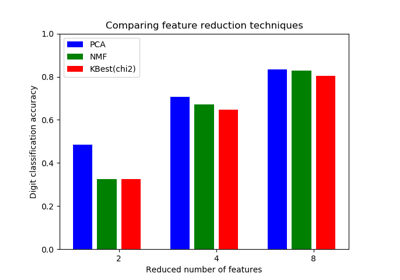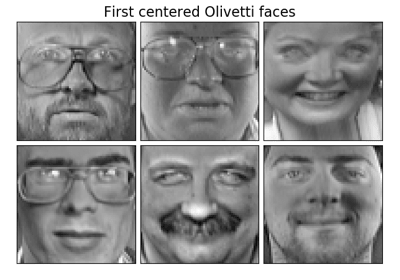/scikit-learn

# sklearn.decomposition.NMF

class sklearn.decomposition.NMF(n_components=None, init=None, solver=’cd’, beta_loss=’frobenius’, tol=0.0001, max_iter=200, random_state=None, alpha=0.0, l1_ratio=0.0, verbose=0, shuffle=False) [source]

Non-Negative Matrix Factorization (NMF)

Find two non-negative matrices (W, H) whose product approximates the non- negative matrix X. This factorization can be used for example for dimensionality reduction, source separation or topic extraction.

The objective function is:

0.5 * ||X - WH||_Fro^2
+ alpha * l1_ratio * ||vec(W)||_1
+ alpha * l1_ratio * ||vec(H)||_1
+ 0.5 * alpha * (1 - l1_ratio) * ||W||_Fro^2
+ 0.5 * alpha * (1 - l1_ratio) * ||H||_Fro^2


Where:

||A||_Fro^2 = \sum_{i,j} A_{ij}^2 (Frobenius norm)
||vec(A)||_1 = \sum_{i,j} abs(A_{ij}) (Elementwise L1 norm)


For multiplicative-update (‘mu’) solver, the Frobenius norm (0.5 * ||X - WH||_Fro^2) can be changed into another beta-divergence loss, by changing the beta_loss parameter.

The objective function is minimized with an alternating minimization of W and H.

Read more in the User Guide.

Parameters: n_components : int or None Number of components, if n_components is not set all features are kept. init : ‘random’ | ‘nndsvd’ | ‘nndsvda’ | ‘nndsvdar’ | ‘custom’ Method used to initialize the procedure. Default: ‘nndsvd’ if n_components < n_features, otherwise random. Valid options: ‘random’: non-negative random matrices, scaled with: sqrt(X.mean() / n_components) ‘nndsvd’: Nonnegative Double Singular Value Decomposition (NNDSVD) initialization (better for sparseness) ‘nndsvda’: NNDSVD with zeros filled with the average of X (better when sparsity is not desired) ‘nndsvdar’: NNDSVD with zeros filled with small random values (generally faster, less accurate alternative to NNDSVDa for when sparsity is not desired) ‘custom’: use custom matrices W and H solver : ‘cd’ | ‘mu’ Numerical solver to use: ‘cd’ is a Coordinate Descent solver. ‘mu’ is a Multiplicative Update solver. New in version 0.17: Coordinate Descent solver. New in version 0.19: Multiplicative Update solver. beta_loss : float or string, default ‘frobenius’ String must be in {‘frobenius’, ‘kullback-leibler’, ‘itakura-saito’}. Beta divergence to be minimized, measuring the distance between X and the dot product WH. Note that values different from ‘frobenius’ (or 2) and ‘kullback-leibler’ (or 1) lead to significantly slower fits. Note that for beta_loss <= 0 (or ‘itakura-saito’), the input matrix X cannot contain zeros. Used only in ‘mu’ solver. New in version 0.19. tol : float, default: 1e-4 Tolerance of the stopping condition. max_iter : integer, default: 200 Maximum number of iterations before timing out. random_state : int, RandomState instance or None, optional, default: None If int, random_state is the seed used by the random number generator; If RandomState instance, random_state is the random number generator; If None, the random number generator is the RandomState instance used by np.random. alpha : double, default: 0. Constant that multiplies the regularization terms. Set it to zero to have no regularization. New in version 0.17: alpha used in the Coordinate Descent solver. l1_ratio : double, default: 0. The regularization mixing parameter, with 0 <= l1_ratio <= 1. For l1_ratio = 0 the penalty is an elementwise L2 penalty (aka Frobenius Norm). For l1_ratio = 1 it is an elementwise L1 penalty. For 0 < l1_ratio < 1, the penalty is a combination of L1 and L2. New in version 0.17: Regularization parameter l1_ratio used in the Coordinate Descent solver. verbose : bool, default=False Whether to be verbose. shuffle : boolean, default: False If true, randomize the order of coordinates in the CD solver. New in version 0.17: shuffle parameter used in the Coordinate Descent solver. components_ : array, [n_components, n_features] Factorization matrix, sometimes called ‘dictionary’. reconstruction_err_ : number Frobenius norm of the matrix difference, or beta-divergence, between the training data X and the reconstructed data WH from the fitted model. n_iter_ : int Actual number of iterations.

#### References

Cichocki, Andrzej, and P. H. A. N. Anh-Huy. “Fast local algorithms for large scale nonnegative matrix and tensor factorizations.” IEICE transactions on fundamentals of electronics, communications and computer sciences 92.3: 708-721, 2009.

Fevotte, C., & Idier, J. (2011). Algorithms for nonnegative matrix factorization with the beta-divergence. Neural Computation, 23(9).

#### Examples

>>> import numpy as np
>>> X = np.array([[1, 1], [2, 1], [3, 1.2], [4, 1], [5, 0.8], [6, 1]])
>>> from sklearn.decomposition import NMF
>>> model = NMF(n_components=2, init='random', random_state=0)
>>> W = model.fit_transform(X)
>>> H = model.components_


#### Methods

 fit(X[, y]) Learn a NMF model for the data X. fit_transform(X[, y, W, H]) Learn a NMF model for the data X and returns the transformed data. get_params([deep]) Get parameters for this estimator. inverse_transform(W) Transform data back to its original space. set_params(**params) Set the parameters of this estimator. transform(X) Transform the data X according to the fitted NMF model
__init__(n_components=None, init=None, solver=’cd’, beta_loss=’frobenius’, tol=0.0001, max_iter=200, random_state=None, alpha=0.0, l1_ratio=0.0, verbose=0, shuffle=False) [source]
fit(X, y=None, **params) [source]

Learn a NMF model for the data X.

Parameters: X : {array-like, sparse matrix}, shape (n_samples, n_features) Data matrix to be decomposed y : Ignored self
fit_transform(X, y=None, W=None, H=None) [source]

Learn a NMF model for the data X and returns the transformed data.

This is more efficient than calling fit followed by transform.

Parameters: X : {array-like, sparse matrix}, shape (n_samples, n_features) Data matrix to be decomposed y : Ignored W : array-like, shape (n_samples, n_components) If init=’custom’, it is used as initial guess for the solution. H : array-like, shape (n_components, n_features) If init=’custom’, it is used as initial guess for the solution. W : array, shape (n_samples, n_components) Transformed data.
get_params(deep=True) [source]

Get parameters for this estimator.

Parameters: deep : boolean, optional If True, will return the parameters for this estimator and contained subobjects that are estimators. params : mapping of string to any Parameter names mapped to their values.
inverse_transform(W) [source]

Transform data back to its original space.

Parameters: W : {array-like, sparse matrix}, shape (n_samples, n_components) Transformed data matrix X : {array-like, sparse matrix}, shape (n_samples, n_features) Data matrix of original shape .. versionadded:: 0.18
set_params(**params) [source]

Set the parameters of this estimator.

The method works on simple estimators as well as on nested objects (such as pipelines). The latter have parameters of the form <component>__<parameter> so that it’s possible to update each component of a nested object.

Returns: self
transform(X) [source]

Transform the data X according to the fitted NMF model

Parameters: X : {array-like, sparse matrix}, shape (n_samples, n_features) Data matrix to be transformed by the model W : array, shape (n_samples, n_components) Transformed data

## Examples using sklearn.decomposition.NMFTopic extraction with Non-negative Matrix Factorization and Latent Dirichlet AllocationSelecting dimensionality reduction with Pipeline and GridSearchCVFaces dataset decompositions